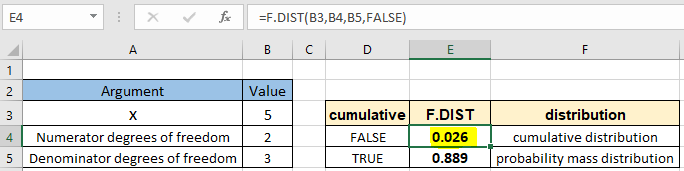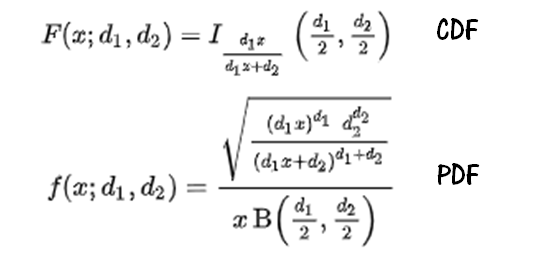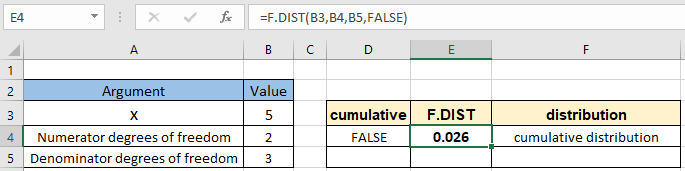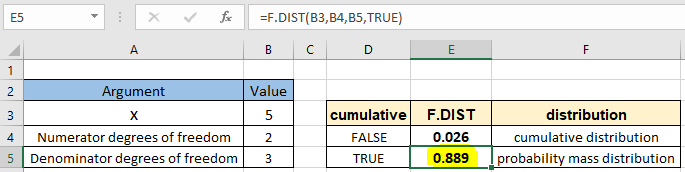# How to use the F.DIST function in ExcelIn this article, we will learn How to use the F.DIST function in Excel.

What is a F-distribution function ?

In probability theory and statistics, the F-distribution, also known as Snedecor's F distribution or the Fisher–Snedecor distribution named after  Ronald Fisher and George W. Snedecor. F-distribution is a continuous probability distribution that arises frequently as the null distribution of a test statistic, most notably in the analysis of variance (ANOVA). Returns the F probability distribution. You can use this function to determine whether two data sets have different degrees of diversity. For example, you can examine the test scores of men and women entering high school, and determine if the variability in the females is different from that found in the males. Mathematically, we can calculate the f distribution function with the formula stated below.Here

1. I is the regularized incomplete beta function.
2. B is the beta function.
3. x is the value at which to evaluate the function.
4. d1 & d2 are the degrees of freedom.

Now you must be wondering what is the degree of freedom (also known as df). Mathematically, the degree of freedom(df) of a distribution is equal to the number of standard normal deviations being summed. But how can we understand the concept of degree of freedom? Degree of freedom is the number of independent possibilities in an event. For example if we toss a coin 100 times and say heads occur 48 times and then we can conclude the tails occured 52 times so the degree of freedom is 1. But if we say on a route of traffic lights (generally has 3 color lights) we want to know the probability of red light in any given sample of time. For this the degree of freedom will be 2 as we will require information for at least 2 color lights. This is too complex to formulate in Excel. So Excel provides a built-in statistical F.DIST function to work as a f-distribution calculator.

F.DIST Function in Excel

F.DIST function built in statistical function that returns the F - probability distribution. It takes the 3 arguments with the type of distribution function (cdf or pdf). Let's understand these arguments one by one listed below with the function syntax.

F.DIST Function syntax:

 =F.DIST(x, deg_freedom1, deg_freedom2, cumulative)

x : value at which to evaluate the function

deg_freedom1 : numerator degrees of freedom

deg_freedom2 : denominator degrees of freedom

cumulative : logical value that determines the form of the function. If cumulative is TRUE, F.DIST returns the cumulative distribution function; if FALSE, it returns the probability density function.

Example :

All of these might be confusing to understand. Let's understand how to use the function using an example. Here we have sample x value with given two degrees of freedom. We need to calculate the cumulative f -  distribution.

Use the formula:

 =F.DIST ( B3, B4, B5, FALSE)The f distribution probability comes out 0.026 or 2.6% for the value at exactly 5.

You can find out the probability value for the f distribution for the value for at least 5 following the same parameters with the formula shown below.

Use the formula:

 =F.DIST ( B3, B4, B5, TRUE)The f distribution probability comes out 0.889 or 88.9% for the value for at least 5.

The F-distribution is a method of obtaining the probabilities of specific sets of events occurring. The F-statistic is often used to assess the significant difference of a theoretical model of the data. Once the F-statistic is calculated, you compare the value to a table of critical values that serve as minimum cutoff values for significance.

Here are all the observational notes using the F.DIST function in Excel
Notes :

1. The function only works with numbers. If any argument other than cumulative is non numeric, the function returns #VALUE! error.
2. The function returns #NUM! Error.
1. If x is negative
2. If the deg_freedom1 is < 1 or >10^10.
3. If the deg_freedom2 is < 1 or >10^10.
3. arguments deg_freedom1 or deg_freedom2 are truncated to integer if not.
4. The cumulative argument can be used with boolean numbers (0 and 1) or (FALSE or TRUE).
5. Value in decimal and value in percentage id the same value in Excel. Convert the value to percentage, if required.
6. You can feed the arguments to the function directly or using the cell reference as explained in the example.

Hope this article about How to use the F.DIST function in Excel is explanatory. Find more articles on statistical formulas and related Excel functions here. If you liked our blogs, share it with your friends on Facebook. And also you can follow us on Twitter and Facebook. We would love to hear from you, do let us know how we can improve, complement or innovate our work and make it better for you. Write to us at info@exceltip.com.

Related Articles :

How to use Excel F.TEST Function in Excel : The F.TEST Function is used to calculate F statistic of two samples in excel internally and returns the two tailed probability of the F statistic under Null Hypothesis.

How To Use Excel T.TEST Function in Excel : The T.TEST function is used to determine the confidence of an analysis in Excel. Mathematically, it is used to know if the mean of the two samples are equal or not. T.TEST function is used to accept or reject the null hypothesis.

How to use the DEVSQ Function in Excel : DEVSQ function is a built-in statistical function to calculate the sum of squared deviations from the mean or average of the given distribution provided.

How to use Excel NORM.DIST Function : Calculate the Z score for the normal cumulative distribution for the pre specified values using the NORMDIST function in Excel.

How to use Excel NORM.INV Function : Calculate the inverse of Z score for the normal cumulative distribution for the pre-specified probability values using the NORM.INV function in Excel.

How to calculate Standard Deviation in Excel : To calculate the standard deviation we have different functions in Excel. The standard deviation is the square root of the variance value but It tells more about the dataset than variance.

How to use the VAR function in Excel : Calculate the variance for the sample dataset in excel using the VAR function in Excel.

Popular Articles :

How to use the IF Function in Excel : The IF statement in Excel checks the condition and returns a specific value if the condition is TRUE or returns another specific value if FALSE.

How to use the VLOOKUP Function in Excel : This is one of the most used and popular functions of excel that is used to lookup value from different ranges and sheets.

How to use the SUMIF Function in Excel : This is another dashboard essential function. This helps you sum up values on specific conditions.

How to use the COUNTIF Function in Excel : Count values with conditions using this amazing function. You don't need to filter your data to count specific values. Countif function is essential to prepare your dashboard.

Terms and Conditions of use

The applications/code on this site are distributed as is and without warranties or liability. In no event shall the owner of the copyrights, or the authors of the applications/code be liable for any loss of profit, any problems or any damage resulting from the use or evaluation of the applications/code.